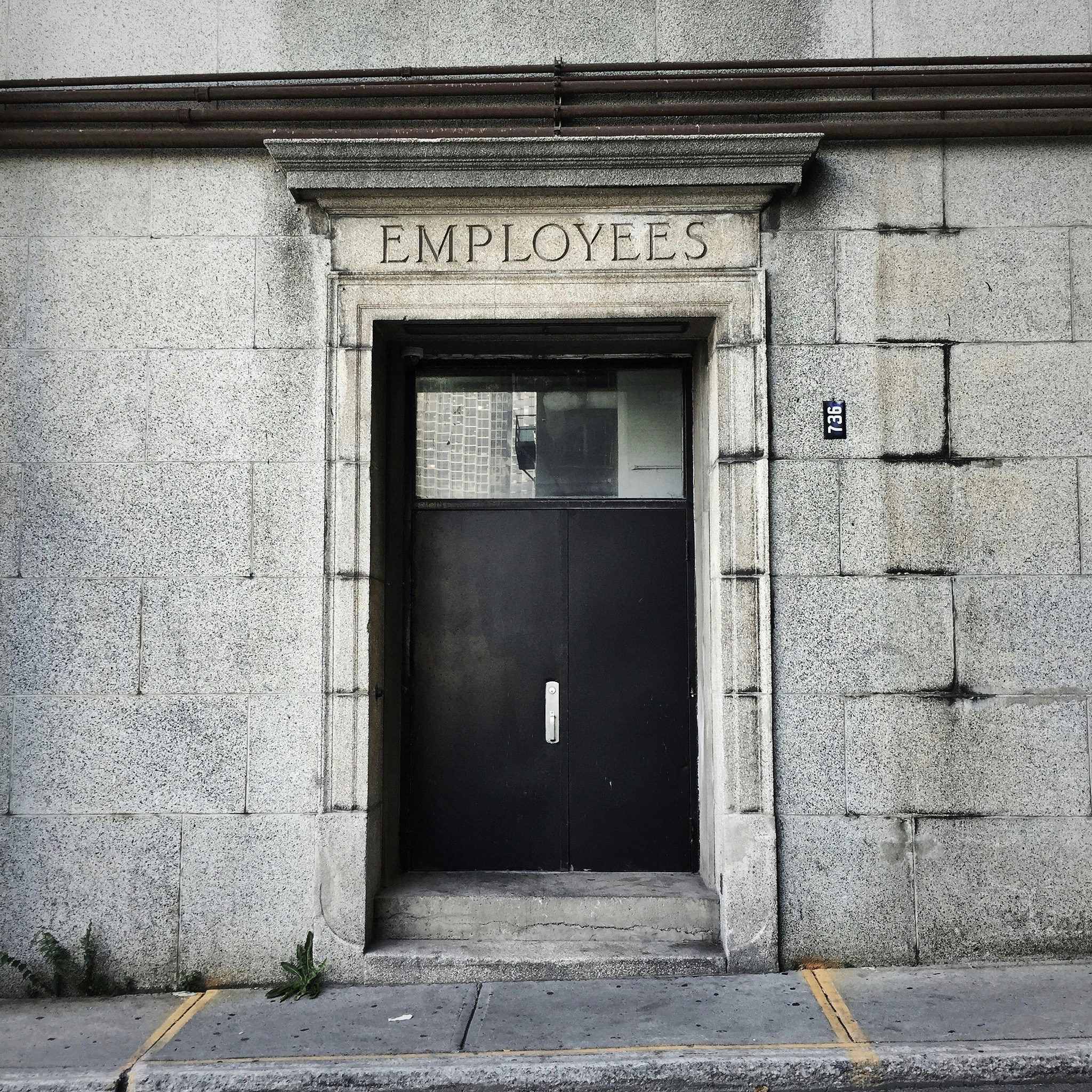# Demystify employee leaving with Machine LearningThis post was originally published by Luke Sun at Towards Data Science

## Creation and Evaluation of Handful of Machine Learning Models for Leave PredictionPhoto by Olivier Collet from Unsplash

`employee_df_cat = pd.get_dummies(employee_df_cat)`
`X_all = pd.concat([X_cat, X_numerical], axis = 1)`
```employee_df[‘Attrition’] = employee_df[‘Attrition’].apply(lambda x: 1 if x == ‘Yes’ else 0)
y = employee_df[‘Attrition’]```
```from sklearn.preprocessing import MinMaxScaler
scaler = MinMaxScaler()
X = scaler.fit_transform(X_all)```
```from sklearn.model_selection import train_test_split
X_train, X_test, y_train, y_test = train_test_split(X, y, test_size = 0.25)```
```oversampler = SMOTE(random_state=0)
X_smote_train, y_smote_train = oversampler.fit_sample(X_train,y_train)```
```from sklearn.linear_model import LogisticRegression
model = LogisticRegression()
model.fit(X_smote_train, y_smote_train)```
```y_pred = model.predict(X_test)
cm = confusion_matrix(y_pred, y_test)
sns.heatmap(cm, annot= True)```
```from sklearn.ensemble import RandomForestClassifier
model = RandomForestClassifier()
model.fit(X_smote_train, y_smote_train)```
```from keras.models import Sequential
from keras.layers import Dense, Dropout
model = Sequential()
model.add(Dense(units = 50, activation = ‘relu’, input_shape = (50, )))
model.add(Dense(units = 500, activation = ‘relu’))
model.add(Dense(units = 500, activation = ‘relu’))
```model.compile(optimizer=’adam’, loss = ‘binary_crossentropy’, metrics = [‘accuracy’])